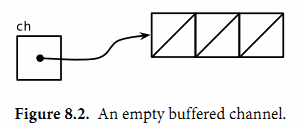## 8.4. Channels

ch := make(chan int) // ch has type 'chan int'


ch <- x  // a send statement
x = <-ch // a receive expression in an assignment statement


Channel還支持close操作，用於關閉channel，隨後對基於該channel的任何發送操作都將導致panic異常。對一個已經被close過的channel之行接收操作依然可以接受到之前已經成功發送的數據；如果channel中已經沒有數據的話講産生一個零值的數據。

close(ch)


ch = make(chan int)    // unbuffered channel
ch = make(chan int, 0) // unbuffered channel
ch = make(chan int, 3) // buffered channel with capacity 3


### 8.4.1. 不帶緩存的Channels

gopl.io/ch8/netcat3
func main() {
conn, err := net.Dial("tcp", "localhost:8000")
if err != nil {
log.Fatal(err)
}
done := make(chan struct{})
go func() {
io.Copy(os.Stdout, conn) // NOTE: ignoring errors
log.Println("done")
done <- struct{}{} // signal the main goroutine
}()
mustCopy(conn, os.Stdin)
conn.Close()
<-done // wait for background goroutine to finish
}


### 8.4.2. 串聯的Channels（Pipeline）

Channels也可以用於將多個goroutine鏈接在一起，一個Channels的輸出作爲下一個Channels的輸入。這種串聯的Channels就是所謂的管道（pipeline）。下面的程序用兩個channels將三個goroutine串聯起來，如圖8.1所示。gopl.io/ch8/pipeline1

func main() {
naturals := make(chan int)
squares := make(chan int)

// Counter
go func() {
for x := 0; ; x++ {
naturals <- x
}
}()

// Squarer
go func() {
for {
x := <-naturals
squares <- x * x
}
}()

// Printer (in main goroutine)
for {
fmt.Println(<-squares)
}
}


close(naturals)


// Squarer
go func() {
for {
x, ok := <-naturals
if !ok {
break // channel was closed and drained
}
squares <- x * x
}
close(squares)
}()


gopl.io/ch8/pipeline2

func main() {
naturals := make(chan int)
squares := make(chan int)

// Counter
go func() {
for x := 0; x < 100; x++ {
naturals <- x
}
close(naturals)
}()

// Squarer
go func() {
for x := range naturals {
squares <- x * x
}
close(squares)
}()

// Printer (in main goroutine)
for x := range squares {
fmt.Println(x)
}
}


### 8.4.3. 單方向的Channel

func counter(out chan int)
func squarer(out, in chan int)
func printer(in chan int)


gopl.io/ch8/pipeline3
func counter(out chan<- int) {
for x := 0; x < 100; x++ {
out <- x
}
close(out)
}

func squarer(out chan<- int, in <-chan int) {
for v := range in {
out <- v * v
}
close(out)
}

func printer(in <-chan int) {
for v := range in {
fmt.Println(v)
}
}

func main() {
naturals := make(chan int)
squares := make(chan int)
go counter(naturals)
go squarer(squares, naturals)
printer(squares)
}


### 8.4.4. 帶緩存的Channels

ch = make(chan string, 3)ch <- "A"
ch <- "B"
ch <- "C"fmt.Println(<-ch) // "A"fmt.Println(cap(ch)) // "3"


fmt.Println(len(ch)) // "2"


fmt.Println(<-ch) // "B"
fmt.Println(<-ch) // "C"


func mirroredQuery() string {
responses := make(chan string, 3)
go func() { responses <- request("asia.gopl.io") }()
go func() { responses <- request("europe.gopl.io") }()
go func() { responses <- request("americas.gopl.io") }()
return <-responses // return the quickest response
}

func request(hostname string) (response string) { /* ... */ }


Channel的緩存也可能影響程序的性能。想象一家蛋糕店有三個廚師，一個烘焙，一個上醣衣，還有一個將每個蛋糕傳遞到它下一個廚師在生産線。在狹小的廚房空間環境，每個廚師在完成蛋糕後必鬚等待下一個廚師已經準備好接受它；這類似於在一個無緩存的channel上進行溝通。